Van der Waals equation of state

The van der Waals equation of state, developed by Johannes Diderik van der Waals  , takes into account two features that are absent in the ideal gas equation of state; the parameter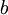$b$ introduces somehow the repulsive behavior between pairs of molecules at short distances, it represents the minimum molar volume of the system, whereas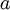$a$ measures the attractive interactions between the molecules. The van der Waals equation of state leads to a liquid-vapor equilibrium at low temperatures, with the corresponding critical point.

Equation of state

The van der Waals equation of state can be written as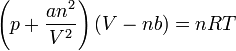$\left(p + \frac{an^2}{V^2}\right)\left(V-nb\right) = nRT$

where:

•$p$ is the pressure,
•$V$ is the volume,
•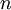$n$ is the number of moles,
•$T$ is the absolute temperature,
•$R$ is the molar gas constant;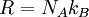$R = N_A k_B$, with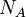$N_A$ being the Avogadro constant and$k_B$ being the Boltzmann constant.
•$a$ and$b$ are constants that introduce the effects of attraction and volume respectively and depend on the substance in question.

Critical point

At the critical point one has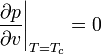$\left.\frac{\partial p}{\partial v}\right|_{T=T_c}=0$, and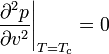$\left.\frac{\partial^2 p}{\partial v^2}\right|_{T=T_c}=0$, leading to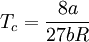$T_c= \frac{8a}{27bR}$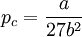$p_c=\frac{a}{27b^2}$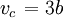$\left.v_c\right.=3b$

along with a critical point compressibility factor of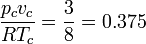$\frac{p_c v_c}{RT_c}= \frac{3}{8} = 0.375$

which then leads to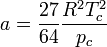$a= \frac{27}{64}\frac{R^2T_c^2}{p_c}$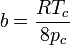$b= \frac{RT_c}{8p_c}$

Virial form

One can re-write the van der Waals equation given above as a virial equation of state as follows: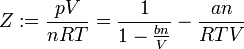$Z := \frac{pV}{nRT} = \frac{1}{1- \frac{bn}{V}} - \frac{an}{RTV}$

Using the well known series expansion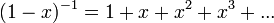$(1-x)^{-1} = 1 + x + x^2 + x^3 + ...$ one can write the first term of the right hand side as :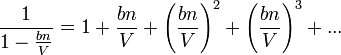$\frac{1}{1- \frac{bn}{V}} = 1 + \frac{bn}{V} + \left( \frac{bn}{V} \right)^2 + \left( \frac{bn}{V} \right)^3 + ...$

Incorporating the second term of the right hand side in its due place leads to: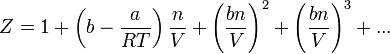$Z = 1 + \left( b -\frac{a}{RT} \right) \frac{n}{V} + \left( \frac{bn}{V} \right)^2 + \left( \frac{bn}{V} \right)^3 + ...$.

From the above one can see that the second virial coefficient corresponds to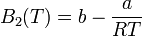$B_{2}(T)= b -\frac{a}{RT}$

and the third virial coefficient is given by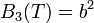$B_{3}(T)= b^2$

Boyle temperature

The Boyle temperature of the van der Waals equation is given by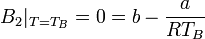$B_2\vert_{T=T_B}=0 = b -\frac{a}{RT_B}$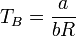$T_B = \frac{a}{bR}$

Dimensionless formulation

If one takes the following reduced quantities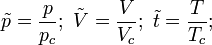$\tilde{p} = \frac{p}{p_c};~ \tilde{V} = \frac{V}{V_c}; ~\tilde{t} = \frac{T}{T_c};$

one arrives at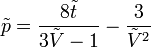$\tilde{p} = \frac{8\tilde{t}}{3\tilde{V} -1} -\frac{3}{\tilde{V}^2}$

The following image is a plot of the isotherms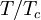$T/T_c$ = 0.85, 0.90, 0.95, 1.0 and 1.05 (from bottom to top) for the van der Waals equation of state:

Critical exponents

The critical exponents of the Van der Waals equation of state place it in the mean field universality class.